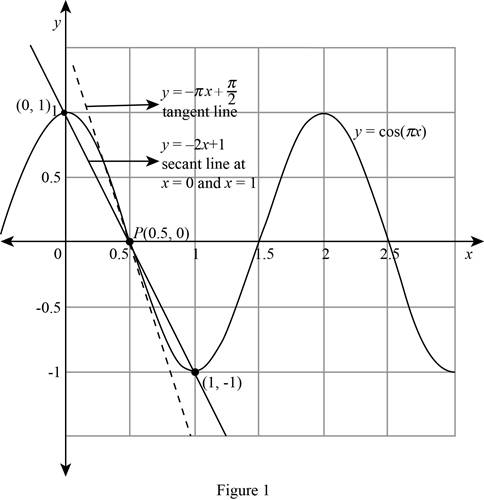# The slope of the secant line PQ for the following values of x.### Single Variable Calculus: Concepts...

4th Edition
James Stewart
Publisher: Cengage Learning
ISBN: 9781337687805### Single Variable Calculus: Concepts...

4th Edition
James Stewart
Publisher: Cengage Learning
ISBN: 9781337687805

#### Solutions

Chapter 2.1, Problem 4E

(a)

To determine

## To find: The slope of the secant line PQ for the following values of x.

Expert Solution

The slope of the secant line PQ for the following values of x is given below:

(i) The slope of the secant line PQ when x=0 is 2.

(ii) The slope of the secant line PQ when x=0.4 is 3.090170.

(iii) The slope of the secant line PQ when x=0.49 is 3.141076.

(iv) The slope of the secant line PQ when x=0.499 is 3.141587.

(v) The slope of the secant line PQ when x=1 is 2.

(vi) The slope of the secant line PQ when x=0.6 is 3.090170.

(vii) The slope of the secant line PQ when x=0.51 is 3.141076.

(viii) The slope of the secant line PQ when x=0.501 is 3.141587.

### Explanation of Solution

Given:

The equation of the curve y=cosπx.

The point P(0.5, 0) lies on the curve y.

The point Q is (x,cosπx).

Calculation:

The slope of the secant line between the points, P(0.5, 0) and Q(x,cosπx) is

mPQ=cosπx0x0.5 (1)

Section (i):

Obtain the slope of the secant line PQ when x=0.

Substitute 0 for x in cosπx.

cosπx=cosπ(0)=1

Substitute Q(x,cosπx)=(0,1) in equation (1).

mPQ=cosπx0x0.5=1000.5=10.5=2

Thus, the slope of the secant line PQ when x=0 is 2.

Section-(ii):

Obtain the slope of the secant line PQ when x=0.4.

Substitute 0.4 for x in cosπx.

cosπx=cosπ(0.4)=0.309016990.309017

Substitute Q(x,cosπx)=(0.4,0.309017) in equation (1).

mPQ=cosπx0x0.5=0.30901700.40.5=0.3090170.13.090170

Thus, the slope of the secant line PQ when x=0.4 is 3.090170.

Section-(iii):

Obtain the slope of the secant line PQ when x=0.49.

Substitute 0.49 for x in cosπx.

cosπx=cosπ(0.49)=0.03141070.031411

Substitute Q(x,cosπx)=(0.49,0.031411) in equation (1).

mPQ=cosπx0x0.5=0.03141100.490.5=0.0314110.013.141076

Thus, the slope of the secant line PQ when x=0.49 is 3.141076.

Section-(iv):

Obtain the slope of the secant line PQ when x=0.499.

Substitute 0.499 for x in cosπx.

cosπx=cosπ(0.499)=0.0031415870.00314159

Substitute Q(x,cosπx)=(0.499,0.00314159) in equation (1).

mPQ=cosπx0x0.5=0.0031415900.4990.5=0.003141590.0013.141587

Thus, the slope of the secant line PQ when x=0.499 is 3.141587.

Section-(v):

Obtain the slope of the secant line PQ when x=1.

Substitute 1 for x in cosπx.

cosπx=cosπ(1)=cosπ=1

Substitute Q(x,cosπx)=(1,1) in equation (1).

mPQ=cosπx0x0.5=1010.5=10.52

Thus, the slope of the secant line PQ when x=1 is 2.

Section-(vi):

Obtain the slope of the secant line PQ when x=0.6.

Substitute 0.6 for x in cosπx.

cosπx=cosπ(0.6)=0.309016990.309017

Substitute Q(x,cosπx)=(0.6,0.309017) in equation (1).

mPQ=cosπx0x0.5=0.30901700.60.5=0.3090170.13.090170

Thus, the slope of the secant line PQ when x=0.6 is 3.090170.

Section-(vii):

Obtain the slope of the secant line PQ when x=0.51.

Substitute 0.49 for x in cosπx.

cosπx=cosπ(0.51)=0.03141070.031411

Substitute Q(x,cosπx)=(0.51,0.031411) in equation (1).

mPQ=cosπx0x0.5=0.03141100.510.5=0.0314110.013.141076

Thus, the slope of the secant line PQ when x=0.51 is 3.141076.

Section-(viii):

Obtain the slope of the secant line PQ when x=0.501.

Substitute 0.501 for x in cosπx.

cosπx=cosπ(0.501)=0.0031415870.00314159

Substitute Q(x,cosπx)=(0.501,0.00314159) in equation (1).

mPQ=cosπx0x0.5=0.0031415900.5010.5=0.003141590.0013.141587

Thus, the slope of the secant line PQ when x=0.501 is 3.141587.

(b)

To determine

### To guess: The slope of the tangent line to the curve at P (0.5, 0).

Expert Solution

The estimated slope of the tangent line to the curve at P (0.5, 0) is π.

### Explanation of Solution

Formula used:

The slope of the tangent line is the limit of the slope of the secant line.

That is, limQP(mPQ)=m (2)

Calculation:

From part (a), the slope of the secant line for many values of x is closer to 1. Thus, the slope mPQ approaches to 3.142 or π .

Substitute mPQ=cosπx0x0.5 in equation (2).

m=limQP(mPQ)=limx0.5(cosπx0x0.5)=(cosπ(0.5)0.50.5)=00

Since 00 is in indeterminate form, apply L’Hospital’s rule and obtain the limit.

limx0.5(cosπx0x0.5)=limx0.5(πsin(πx))=(πsin(π(0.5)))=π(1)=π

Thus, the estimated slope of the tangent line to the curve at P (0.5, 0) is π.

(c)

To determine

### To find: The equation of the tangent line to the curve at P(0.5, 0).

Expert Solution

The equation of the tangent line to the curve at P(0.5, 0) is y=πx+12π_

### Explanation of Solution

Formula used:

The equation of the tangent line to the curve y=f(x) at the point (x1,y1):

yy1=m(xx1) (3)

Calculation:

Substitute m=π and (x1,y1)=(0.5,0) in equation (3).

y0=π(x0.5)y=πx+0.5π=πx+12π

Thus, the equation of the tangent line to the curve at P(0.5,0) is y=πx+12π_.

(d)

To determine

Expert Solution

### Explanation of Solution

Formula used:

Slope-intercept formula: yy1=mPQ(xx1)

Calculation:

Obtain the secant line at x=0.

The slope of the secant line PQ when x=0 is 2.

Use the slope-point intercept formula to find a equation of the secant line.

Substitute (x1,y1)=(0,1) and mPQ=2 in the Slope-point intercept formula.

y1=2(x0)y1=2xy=2x+1

Thus, the equation of the secant line at x=0 is y=2x+1.

Obtain the secant line at x=1.

The slope of the secant line PQ when x=1 is 2.

Use the slope-point intercept formula to find a equation of the secant line.

Substitute (x1,y1)=(1,1) and mPQ=2 in the Slope-point intercept formula.

y(1)=2(x1)y+1=2x+2y=2x+21=2x+1

Thus, the equation of the secant line at x=1 is y=2x+1.

Note that the equation of the secant line at x=0 and x=1 are the same.

Draw the curve y=cosπx , two of the secant lines y=2x+1, and the tangent line y=πx+π2 as shown below in Figure 1.Thus, the required sketch is obtained.

### Have a homework question?

Subscribe to bartleby learn! Ask subject matter experts 30 homework questions each month. Plus, you’ll have access to millions of step-by-step textbook answers!